import pandas as pd
import numpy as np
import matplotlib.pyplot as plt
import seaborn as sns

plt.rcParams['figure.figsize'] = (10, 5)
plt.style.use('fivethirtyeight')


## Seasonal time series

• Seasonal data
• Has predictable and repeated patterns
• Repeats after any amount of time
• Seasonal decomposition
• time series = trend + seasonal + redisdual

### Seasonal decompose

You can think of a time series as being composed of trend, seasonal and residual components. This can be a good way to think about the data when you go about modeling it. If you know the period of the time series you can decompose it into these components.

In this exercise you will decompose a time series showing the monthly milk production per cow in the USA. This will give you a clearer picture of the trend and the seasonal cycle. Since the data is monthly you will guess that the seasonality might be 12 time periods, however this won't always be the case.

milk_production = pd.read_csv('./dataset/milk_production.csv', index_col='date', parse_dates=True)
milk_production = milk_production.asfreq('MS')

pounds_per_cow
date
1962-01-01 589.0
1962-02-01 561.0
1962-03-01 640.0
1962-04-01 656.0
1962-05-01 727.0
from statsmodels.tsa.seasonal import seasonal_decompose

decomp = seasonal_decompose(milk_production['pounds_per_cow'], period=12)

# Plot decomposition
decomp.plot();
plt.tight_layout();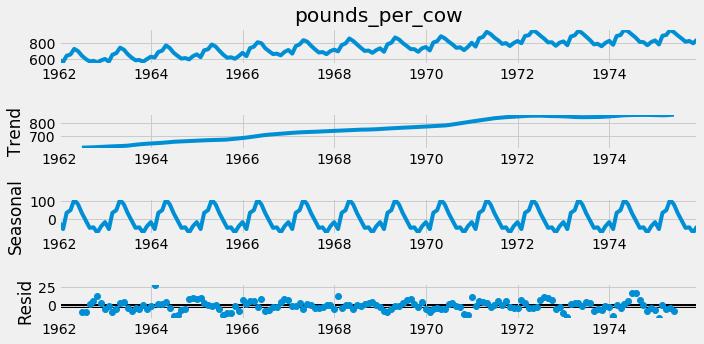### Seasonal ACF and PACF

Below is a time series showing the estimated number of water consumers in London. By eye you can't see any obvious seasonal pattern, however your eyes aren't the best tools you have.

water = pd.read_csv('./dataset/water.csv', index_col='date', parse_dates=True)
water = water.asfreq('MS')

water_consumers
date
1983-01-01 24963
1983-02-01 27380
1983-03-01 32588
1983-04-01 25511
1983-05-01 32313
water.plot();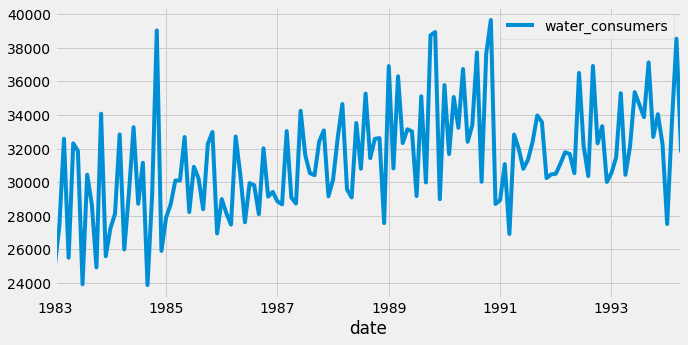In this exercise you will use the ACF and PACF to test this data for seasonality. You can see from the plot above that the time series isn't stationary, so you should probably detrend it. You will detrend it by subtracting the moving average. Remember that you could use a window size of any value bigger than the likely period.

from statsmodels.graphics.tsaplots import plot_acf

# Create figure and subplot
fig, ax1 = plt.subplots()

# Plot the ACF on ax1
plot_acf(water['water_consumers'], lags=25, zero=False, ax=ax1);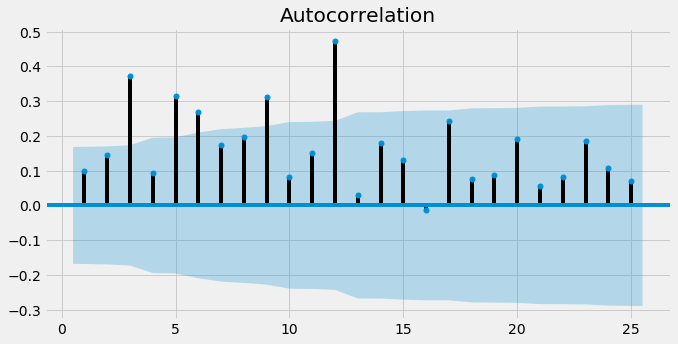water_2 = water - water.rolling(15).mean()

# Drop the NaN values
water_2 = water_2.dropna()

# Create figure and subplots
fig, ax1 = plt.subplots()

# Plot the ACF
plot_acf(water_2['water_consumers'], lags=25, zero=False, ax=ax1);Based on this figure, 12 time steps is the time period of the seasonal component.

## SARIMA models

• Seasonal ARIMA = SARIMA $$\text{SARIMA}(p, d, q)(P, D, Q)_S$$
• Non-seasonal orders
• p: autoregressive order
• d: differencing order
• q: moving average order
• Seasonal orders
• P: seasonal autoregressive order
• D: seasonal differencing order
• Q: seasonal moving average order
• S: Number of time steps per cycle
• The SARIMA model

• ARIMA(2, 0, 1) model: $$y_t = a_1 y_{t-1} + a_2 y_{t-2} + m_1 \epsilon_{t-1} + \epsilon_t$$
• $\text{SARIMA}(0,0,0)(2, 0, 1)_7$ model: $$y_t = a_7 y_{t-7} + a_{14} y_{t-14} + m_y \epsilon_{t-7} + \epsilon_t$$
• Seasonal differencing

• Subtract the time series value of one season ago $$\Delta y_t = y_t - y_{t-S}$$

### Fitting SARIMA models

Fitting SARIMA models is the beginning of the end of this journey into time series modeling.

It is important that you get to know your way around the SARIMA model orders and so that's what you will focus on here.

In this exercise, you will practice fitting different SARIMA models to a set of time series.

df1 = pd.read_csv('./dataset/df1.csv', index_col=0, parse_dates=True)

df1 = df1.asfreq('d')
df2 = df2.asfreq('d')
df3 = df3.asfreq('d')

from statsmodels.tsa.statespace.sarimax import SARIMAX

# Create a SARIMAX model
model = SARIMAX(df1, order=(1, 0, 0), seasonal_order=(1, 1, 0, 7))

# Fit the model
results = model.fit()

# Print the results summary
results.summary()

Dep. Variable: No. Observations: Y 90 SARIMAX(1, 0, 0)x(1, 1, 0, 7) -556.243 Tue, 16 Jun 2020 1118.486 15:34:16 1125.742 01-01-2013 1121.401 - 03-31-2013 opg
coef std err z P>|z| [0.025 0.975] 0.1032 0.103 1.002 0.316 -0.099 0.305 0.2781 0.104 2.665 0.008 0.074 0.483 3.858e+04 7224.680 5.340 0.000 2.44e+04 5.27e+04
 Ljung-Box (Q): Jarque-Bera (JB): 41.76 1.69 0.39 0.43 1.35 -0.15 0.43 2.37

Warnings:
 Covariance matrix calculated using the outer product of gradients (complex-step).
model = SARIMAX(df2, order=(2, 1, 1), seasonal_order=(1, 0, 0, 4))

# Fit the model
results = model.fit()

# Print the results summary
results.summary()

Dep. Variable: No. Observations: Y 80 SARIMAX(2, 1, 1)x(1, 0, [], 4) -560.340 Tue, 16 Jun 2020 1130.679 15:34:17 1142.526 01-01-2013 1135.426 - 03-21-2013 opg
coef std err z P>|z| [0.025 0.975] 0.2701 0.162 1.672 0.095 -0.047 0.587 0.5015 0.110 4.560 0.000 0.286 0.717 -0.4271 0.178 -2.401 0.016 -0.776 -0.078 0.1075 0.127 0.847 0.397 -0.141 0.356 8.45e+04 1.63e+04 5.178 0.000 5.25e+04 1.16e+05
 Ljung-Box (Q): Jarque-Bera (JB): 30.8 0.95 0.85 0.62 0.6 -0.07 0.2 2.48

Warnings:
 Covariance matrix calculated using the outer product of gradients (complex-step).
model = SARIMAX(df3, order=(1, 1, 0), seasonal_order=(0, 1, 1, 12))

# Fit the model
results = model.fit()

# Print the results summary
results.summary()

Dep. Variable: No. Observations: Y 100 SARIMAX(1, 1, 0)x(0, 1, , 12) -521.376 Tue, 16 Jun 2020 1048.752 15:34:17 1056.149 01-01-2013 1051.730 - 04-10-2013 opg
coef std err z P>|z| [0.025 0.975] 0.4236 0.090 4.719 0.000 0.248 0.600 -0.0898 0.116 -0.776 0.438 -0.317 0.137 9347.1462 1407.490 6.641 0.000 6588.516 1.21e+04
 Ljung-Box (Q): Jarque-Bera (JB): 33.11 0.02 0.77 0.99 0.77 0.02 0.48 3.05

Warnings:
 Covariance matrix calculated using the outer product of gradients (complex-step).

### Choosing SARIMA order

In this exercise you will find the appropriate model order for a new set of time series. This is monthly series of the number of employed persons in Australia (in thousands). The seasonal period of this time series is 12 months.

You will create non-seasonal and seasonal ACF and PACF plots and use the table below to choose the appropriate model orders.

AR(p) MA(q) ARMA(p, q)
ACF Tails off Cuts off after lag q Tails off
PACF Cuts off after lag p Tails off Tails off
aus_employment = pd.read_csv('./dataset/aus_employment.csv', index_col='date', parse_dates=True)
aus_employment = aus_employment.asfreq('MS')

people_employed
date
1978-01-01 5985.7
1978-02-01 6040.6
1978-03-01 6054.2
1978-04-01 6038.3
1978-05-01 6031.3
from statsmodels.graphics.tsaplots import plot_pacf

# Take the first and seasonal differences and drop NaNs
aus_employment_diff = aus_employment.diff().diff(12).dropna()

# Create the figure
fig, (ax1, ax2) = plt.subplots(2,1,figsize=(8,6))

# Plot the ACF on ax1
plot_acf(aus_employment_diff, lags=11, zero=False, ax=ax1);

# Plot the PACF on ax2
plot_pacf(aus_employment_diff, lags=11, zero=False, ax=ax2);
plt.tight_layout();lags = [12, 24, 36, 48, 60]

# Create the figure
fig, (ax1, ax2) = plt.subplots(2,1,figsize=(8,6))

# Plot the ACF on ax1
plot_acf(aus_employment_diff, lags=lags, zero=False, ax=ax1);

# Plot the PACF on ax2
plot_pacf(aus_employment_diff, lags=lags, zero=False, ax=ax2);
plt.tight_layout();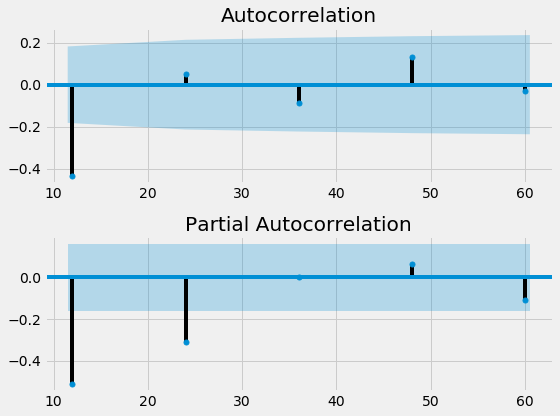The non-seasonal ACF doesn't show any of the usual patterns of MA, AR or ARMA models so we choose none of these. The Seaosnal ACF and PACF look like an MA(1) model. : $\text{SARIMAX}(0,1,0)(0,1,1)_{12}$

### SARIMA vs ARIMA forecasts

In this exercise, you will see the effect of using a SARIMA model instead of an ARIMA model on your forecasts of seasonal time series.

Two models, an ARIMA(3,1,2) and a SARIMA(0,1,1)(1,1,1)12, have been fit to the Wisconsin employment time series. These were the best ARIMA model and the best SARIMA model available according to the AIC.

In the exercise you will use these two models to make dynamic future forecast for 25 months and plot these predictions alongside held out data for this period, wisconsin_test.

wisconsin_test = pd.read_csv('./dataset/wisconsin_test.csv', index_col='date', parse_dates=True)
wisconsin_test = wisconsin_test.asfreq('MS')
dates = wisconsin_test.index

number_in_employment
date
1973-10-01 374.5
1973-11-01 380.2
1973-12-01 384.6
1974-01-01 360.6
1974-02-01 354.4
model = SARIMAX(wisconsin_test, order=(3, 1, 2), trend='c',
enforce_stationarity=True, enforce_invertibility=True)

arima_results = model.fit()

/home/chanseok/anaconda3/lib/python3.7/site-packages/statsmodels/base/model.py:568: ConvergenceWarning: Maximum Likelihood optimization failed to converge. Check mle_retvals
"Check mle_retvals", ConvergenceWarning)

model = SARIMAX(wisconsin_test, order=(0, 1, 1), seasonal_order=(1, 1, 1, 12), trend='c',
enforce_stationarity=True, enforce_invertibility=True)

sarima_results = model.fit()

/home/chanseok/anaconda3/lib/python3.7/site-packages/statsmodels/tsa/statespace/sarimax.py:868: UserWarning: Too few observations to estimate starting parameters for seasonal ARMA. All parameters except for variances will be set to zeros.
' zeros.' % warning_description)
/home/chanseok/anaconda3/lib/python3.7/site-packages/statsmodels/base/model.py:568: ConvergenceWarning: Maximum Likelihood optimization failed to converge. Check mle_retvals
"Check mle_retvals", ConvergenceWarning)

arima_results.specification

{'seasonal_periods': 0,
'measurement_error': False,
'time_varying_regression': False,
'simple_differencing': False,
'enforce_stationarity': True,
'enforce_invertibility': True,
'hamilton_representation': False,
'concentrate_scale': False,
'trend_offset': 1,
'order': (3, 1, 2),
'seasonal_order': (0, 0, 0, 0),
'k_diff': 1,
'k_seasonal_diff': 0,
'k_ar': 3,
'k_ma': 2,
'k_seasonal_ar': 0,
'k_seasonal_ma': 0,
'k_ar_params': 3,
'k_ma_params': 2,
'trend': 'c',
'k_trend': 1,
'k_exog': 0,
'mle_regression': False,
'state_regression': False}
sarima_results.specification

{'seasonal_periods': 12,
'measurement_error': False,
'time_varying_regression': False,
'simple_differencing': False,
'enforce_stationarity': True,
'enforce_invertibility': True,
'hamilton_representation': False,
'concentrate_scale': False,
'trend_offset': 1,
'order': (0, 1, 1),
'seasonal_order': (1, 1, 1, 12),
'k_diff': 1,
'k_seasonal_diff': 1,
'k_ar': 0,
'k_ma': 1,
'k_seasonal_ar': 12,
'k_seasonal_ma': 12,
'k_ar_params': 0,
'k_ma_params': 1,
'trend': 'c',
'k_trend': 1,
'k_exog': 0,
'mle_regression': False,
'state_regression': False}
arima_pred = arima_results.get_forecast(25)
arima_mean = arima_pred.predicted_mean

# Create SARIMA mean forecast
sarima_pred = sarima_results.get_forecast(25)
sarima_mean = sarima_pred.predicted_mean

# Plot mean ARIMA and SARIMA predictions and observed
plt.plot(dates, sarima_mean, label='SARIMA');
plt.plot(dates, arima_mean, label='ARIMA');
plt.plot(wisconsin_test, label='observed');
plt.legend();## Automation and saving

• pmdarima packages for Automated model selection

### Automated model selection

The pmdarima package is a powerful tool to help you choose the model orders. You can use the information you already have from the identification step to narrow down the model orders which you choose by automation.

Remember, although automation is powerful, it can sometimes make mistakes that you wouldn't. It is hard to guess how the input data could be imperfect and affect the test scores.

In this exercise you will use the pmdarima package to automatically choose model orders for some time series datasets.

import pmdarima as pm

# Create auto_arima model
model1 = pm.auto_arima(df1,
seasonal=True, m=7,
d=0, D=1,
max_p=2, max_q=2,
trace=True,
error_action='ignore',
suppress_warnings=True)

# Print model summary
print(model1.summary())

Performing stepwise search to minimize aic
Fit ARIMA(2,0,2)x(1,1,1,7) [intercept=True]; AIC=1125.412, BIC=1144.763, Time=0.506 seconds
Fit ARIMA(0,0,0)x(0,1,0,7) [intercept=True]; AIC=1124.433, BIC=1129.271, Time=0.009 seconds
Fit ARIMA(1,0,0)x(1,1,0,7) [intercept=True]; AIC=1120.434, BIC=1130.109, Time=0.119 seconds
Fit ARIMA(0,0,1)x(0,1,1,7) [intercept=True]; AIC=1122.218, BIC=1131.893, Time=0.075 seconds
Fit ARIMA(0,0,0)x(0,1,0,7) [intercept=False]; AIC=1122.596, BIC=1125.015, Time=0.006 seconds
Fit ARIMA(1,0,0)x(0,1,0,7) [intercept=True]; AIC=1125.048, BIC=1132.305, Time=0.016 seconds
Fit ARIMA(1,0,0)x(2,1,0,7) [intercept=True]; AIC=1119.909, BIC=1132.004, Time=0.233 seconds
Fit ARIMA(1,0,0)x(2,1,1,7) [intercept=True]; AIC=1121.791, BIC=1136.304, Time=0.209 seconds
Fit ARIMA(1,0,0)x(1,1,1,7) [intercept=True]; AIC=1120.954, BIC=1133.049, Time=0.157 seconds
Fit ARIMA(0,0,0)x(2,1,0,7) [intercept=True]; AIC=1119.066, BIC=1128.742, Time=0.166 seconds
Fit ARIMA(0,0,0)x(1,1,0,7) [intercept=True]; AIC=1119.294, BIC=1126.550, Time=0.092 seconds
Fit ARIMA(0,0,0)x(2,1,1,7) [intercept=True]; AIC=1120.813, BIC=1132.907, Time=0.173 seconds
Fit ARIMA(0,0,0)x(1,1,1,7) [intercept=True]; AIC=1120.054, BIC=1129.729, Time=0.090 seconds
Fit ARIMA(0,0,1)x(2,1,0,7) [intercept=True]; AIC=1119.937, BIC=1132.031, Time=0.126 seconds
Fit ARIMA(1,0,1)x(2,1,0,7) [intercept=True]; AIC=1120.607, BIC=1135.120, Time=0.397 seconds
Near non-invertible roots for order (1, 0, 1)(2, 1, 0, 7); setting score to inf (at least one inverse root too close to the border of the unit circle: 1.000)
Total fit time: 2.380 seconds
SARIMAX Results
===============================================================================
Dep. Variable:                       y   No. Observations:                   90
Model:             SARIMAX(2, 1, 0, 7)   Log Likelihood                -555.533
Date:                 Tue, 16 Jun 2020   AIC                           1119.066
Time:                         15:34:55   BIC                           1128.742
Sample:                              0   HQIC                          1122.953
- 90
Covariance Type:                   opg
==============================================================================
coef    std err          z      P>|z|      [0.025      0.975]
------------------------------------------------------------------------------
intercept     -5.3485     22.658     -0.236      0.813     -49.757      39.060
ar.S.L7        0.2326      0.116      2.014      0.044       0.006       0.459
ar.S.L14       0.1716      0.127      1.356      0.175      -0.077       0.420
sigma2       3.79e+04   7066.970      5.363      0.000     2.4e+04    5.18e+04
===================================================================================
Ljung-Box (Q):                       33.73   Jarque-Bera (JB):                 1.88
Prob(Q):                              0.75   Prob(JB):                         0.39
Heteroskedasticity (H):               1.37   Skew:                            -0.24
Prob(H) (two-sided):                  0.41   Kurtosis:                         2.44
===================================================================================

Warnings:
 Covariance matrix calculated using the outer product of gradients (complex-step).

model2 = pm.auto_arima(df2,
seasonal=False,
d=1,
trend='c',
max_p=2, max_q=2,
trace=True,
error_action='ignore',
suppress_warnings=True)

# Print model summary
print(model2.summary())

Performing stepwise search to minimize aic
Fit ARIMA(2,1,2)x(0,0,0,0) [intercept=True]; AIC=1131.956, BIC=1146.173, Time=0.092 seconds
Fit ARIMA(0,1,0)x(0,0,0,0) [intercept=True]; AIC=1153.963, BIC=1158.702, Time=0.005 seconds
Fit ARIMA(1,1,0)x(0,0,0,0) [intercept=True]; AIC=1155.355, BIC=1162.464, Time=0.028 seconds
Fit ARIMA(0,1,1)x(0,0,0,0) [intercept=True]; AIC=1155.747, BIC=1162.855, Time=0.011 seconds
Fit ARIMA(0,1,0)x(0,0,0,0) [intercept=False]; AIC=1153.963, BIC=1158.702, Time=0.005 seconds
Fit ARIMA(1,1,2)x(0,0,0,0) [intercept=True]; AIC=1136.103, BIC=1147.950, Time=0.114 seconds
Fit ARIMA(2,1,1)x(0,0,0,0) [intercept=True]; AIC=1130.992, BIC=1142.840, Time=0.058 seconds
Fit ARIMA(1,1,1)x(0,0,0,0) [intercept=True]; AIC=1149.020, BIC=1158.498, Time=0.087 seconds
Fit ARIMA(2,1,0)x(0,0,0,0) [intercept=True]; AIC=1132.526, BIC=1142.003, Time=0.067 seconds
Total fit time: 0.469 seconds
SARIMAX Results
==============================================================================
Dep. Variable:                      y   No. Observations:                   80
Model:               SARIMAX(2, 1, 1)   Log Likelihood                -560.496
Date:                Tue, 16 Jun 2020   AIC                           1130.992
Time:                        15:34:55   BIC                           1142.840
Sample:                             0   HQIC                          1135.739
- 80
Covariance Type:                  opg
==============================================================================
coef    std err          z      P>|z|      [0.025      0.975]
------------------------------------------------------------------------------
intercept     -9.5411     20.071     -0.475      0.635     -48.879      29.797
ar.L1          0.2744      0.148      1.849      0.064      -0.016       0.565
ar.L2          0.5383      0.098      5.503      0.000       0.347       0.730
ma.L1         -0.4587      0.159     -2.886      0.004      -0.770      -0.147
sigma2      8.558e+04   1.65e+04      5.197      0.000    5.33e+04    1.18e+05
===================================================================================
Ljung-Box (Q):                       30.31   Jarque-Bera (JB):                 0.85
Prob(Q):                              0.87   Prob(JB):                         0.66
Heteroskedasticity (H):               0.60   Skew:                            -0.09
Prob(H) (two-sided):                  0.20   Kurtosis:                         2.53
===================================================================================

Warnings:
 Covariance matrix calculated using the outer product of gradients (complex-step).

model3 = pm.auto_arima(df3,
seasonal=True, m=7,
d=1, D=1,
start_p=1, start_q=1,
max_p=1, max_q=1,
max_P=1, max_Q=1,
trace=True,
error_action='ignore',
suppress_warnings=True)

# Print model summary
print(model3.summary())

Performing stepwise search to minimize aic
Fit ARIMA(1,1,1)x(1,1,1,7) [intercept=True]; AIC=1566.379, BIC=1581.510, Time=0.427 seconds
Fit ARIMA(0,1,0)x(0,1,0,7) [intercept=True]; AIC=1595.826, BIC=1600.870, Time=0.007 seconds
Fit ARIMA(1,1,0)x(1,1,0,7) [intercept=True]; AIC=1585.203, BIC=1595.290, Time=0.093 seconds
Fit ARIMA(0,1,1)x(0,1,1,7) [intercept=True]; AIC=1572.745, BIC=1582.832, Time=0.218 seconds
Near non-invertible roots for order (0, 1, 1)(0, 1, 1, 7); setting score to inf (at least one inverse root too close to the border of the unit circle: 0.999)
Fit ARIMA(0,1,0)x(0,1,0,7) [intercept=False]; AIC=1593.897, BIC=1596.419, Time=0.009 seconds
Fit ARIMA(1,1,1)x(0,1,1,7) [intercept=True]; AIC=1558.168, BIC=1570.777, Time=0.298 seconds
Near non-invertible roots for order (1, 1, 1)(0, 1, 1, 7); setting score to inf (at least one inverse root too close to the border of the unit circle: 1.000)
Fit ARIMA(1,1,1)x(1,1,0,7) [intercept=True]; AIC=1565.768, BIC=1578.377, Time=0.259 seconds
Near non-invertible roots for order (1, 1, 1)(1, 1, 0, 7); setting score to inf (at least one inverse root too close to the border of the unit circle: 1.000)
Fit ARIMA(1,1,1)x(0,1,0,7) [intercept=True]; AIC=1579.028, BIC=1589.116, Time=0.107 seconds
Near non-invertible roots for order (1, 1, 1)(0, 1, 0, 7); setting score to inf (at least one inverse root too close to the border of the unit circle: 0.994)
Fit ARIMA(0,1,1)x(1,1,1,7) [intercept=True]; AIC=1571.169, BIC=1583.778, Time=0.323 seconds
Fit ARIMA(1,1,0)x(1,1,1,7) [intercept=True]; AIC=1570.775, BIC=1583.384, Time=0.260 seconds
Near non-invertible roots for order (1, 1, 0)(1, 1, 1, 7); setting score to inf (at least one inverse root too close to the border of the unit circle: 0.993)
Fit ARIMA(0,1,0)x(1,1,1,7) [intercept=True]; AIC=1573.132, BIC=1583.219, Time=0.244 seconds
Near non-invertible roots for order (0, 1, 0)(1, 1, 1, 7); setting score to inf (at least one inverse root too close to the border of the unit circle: 0.997)
Total fit time: 2.248 seconds
SARIMAX Results
=========================================================================================
Dep. Variable:                                 y   No. Observations:                  100
Model:             SARIMAX(1, 1, 1)x(0, 1, 1, 7)   Log Likelihood                -774.084
Date:                           Tue, 16 Jun 2020   AIC                           1558.168
Time:                                   15:34:58   BIC                           1570.777
Sample:                                        0   HQIC                          1563.257
- 100
Covariance Type:                             opg
==============================================================================
coef    std err          z      P>|z|      [0.025      0.975]
------------------------------------------------------------------------------
intercept      1.7464      2.134      0.819      0.413      -2.435       5.928
ar.L1          0.4239      0.127      3.342      0.001       0.175       0.672
ma.L1         -1.0000      0.250     -4.004      0.000      -1.489      -0.510
ma.S.L7       -0.9382      0.231     -4.056      0.000      -1.392      -0.485
sigma2      1.008e+06    2.6e-07   3.88e+12      0.000    1.01e+06    1.01e+06
===================================================================================
Ljung-Box (Q):                      272.00   Jarque-Bera (JB):                11.30
Prob(Q):                              0.00   Prob(JB):                         0.00
Heteroskedasticity (H):               1.07   Skew:                             0.85
Prob(H) (two-sided):                  0.86   Kurtosis:                         2.84
===================================================================================

Warnings:
 Covariance matrix calculated using the outer product of gradients (complex-step).
 Covariance matrix is singular or near-singular, with condition number 3.73e+29. Standard errors may be unstable.


### Saving and updating models

Once you have gone through the steps of the Box-Jenkins method and arrived at a model you are happy with, you will want to be able to save that model and also to incorporate new measurements when they are available. This is key part of putting the model into production.

In this exercise you will save a freshly trained model to disk, then reload it to update it with new data.

import joblib

# Set model name
filename='candy_model.pkl'

# Pickle it
joblib.dump(model1, filename)

# Load the model back in

# Update the model


## SARIMA and Box-Jenkins

• Box-Jenkins with seasonal data
• Determine if time series is seasonal
• Find seasonal period
• Find transforms to make data stationary
• Seasonal and non-seasonal differencing
• Other transforms

### SARIMA model diagnostics

Usually the next step would be to find the order of differencing and other model orders. However, this time it's already been done for you. The time series is best fit by a $\text{SARIMA}(1, 1, 1)(0, 1, 1)_{12}$ model with an added constant.

In this exercise you will make sure that this is a good model by first fitting it using the SARIMAX class and going through the normal model diagnostics procedure.

co2 = pd.read_csv('./dataset/co2.csv', index_col='date', parse_dates=True)
co2 = co2.asfreq('MS')

CO2_ppm
date
1958-03-01 315.71
1958-04-01 317.45
1958-05-01 317.50
1958-06-01 317.10
1958-07-01 315.86
model = SARIMAX(co2, order=(1, 1, 1), seasonal_order=(0, 1, 1, 12), trend='c')

# Fit model
results = model.fit()

results.summary()

Dep. Variable: No. Observations: CO2_ppm 727 SARIMAX(1, 1, 1)x(0, 1, 1, 12) -179.718 Tue, 16 Jun 2020 369.437 15:35:01 392.291 03-01-1958 378.263 - 09-01-2018 opg
coef std err z P>|z| [0.025 0.975] 0.0017 0.001 2.809 0.005 0.001 0.003 0.2411 0.078 3.072 0.002 0.087 0.395 -0.6079 0.065 -9.296 0.000 -0.736 -0.480 -0.8820 0.019 -45.520 0.000 -0.920 -0.844 0.0944 0.005 20.750 0.000 0.085 0.103
 Ljung-Box (Q): Jarque-Bera (JB): 47 6.19 0.21 0.05 1.13 0 0.33 3.46

Warnings:
 Covariance matrix calculated using the outer product of gradients (complex-step).
results.plot_diagnostics(figsize=(20, 15));
plt.tight_layout();### SARIMA forecast

In the previous exercise you confirmed that a SARIMA (1,1,1) x (0,1,1)12 model was a good fit to the CO2 time series by using diagnostic checking.

Now its time to put this model into practice to make future forecasts. Climate scientists tell us that we have until 2030 to drastically reduce our CO2 emissions or we will face major societal challenges.

In this exercise, you will forecast the CO2 time series up to the year 2030 to find the CO2 levels if we continue emitting as usual.

forecast_object = results.get_forecast(136)

# Extract predicted mean attribute
mean = forecast_object.predicted_mean

# Calculate the confidence intervals
conf_int = forecast_object.conf_int()

# Extract the forecast dates
dates = mean.index

plt.figure()

# Plot past CO2 levels
plt.plot(co2.index, co2, label='past');

# Plot the prediction means as line
plt.plot(dates, mean, label='predicted');

# Shade between the confidence intervals
plt.fill_between(dates, conf_int.loc[:, 'lower CO2_ppm'],
conf_int.loc[:, 'upper CO2_ppm'], alpha=0.4);

# Plot legend
plt.legend();
plt.savefig('../images/co2_predicted.png')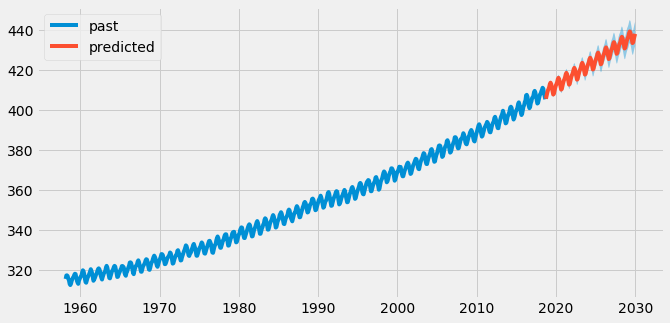print(mean.iloc[-1])

# Print last confidence interval
print(conf_int.iloc[-1])

438.2001450227593
lower CO2_ppm    432.118155
upper CO2_ppm    444.282135
Name: 2030-01-01 00:00:00, dtype: float64


### The SARIMAX model

S - seasonal\ AR - AutoRegressive\ I - Integrated\ MA - Moving Average\ X - Exogenous